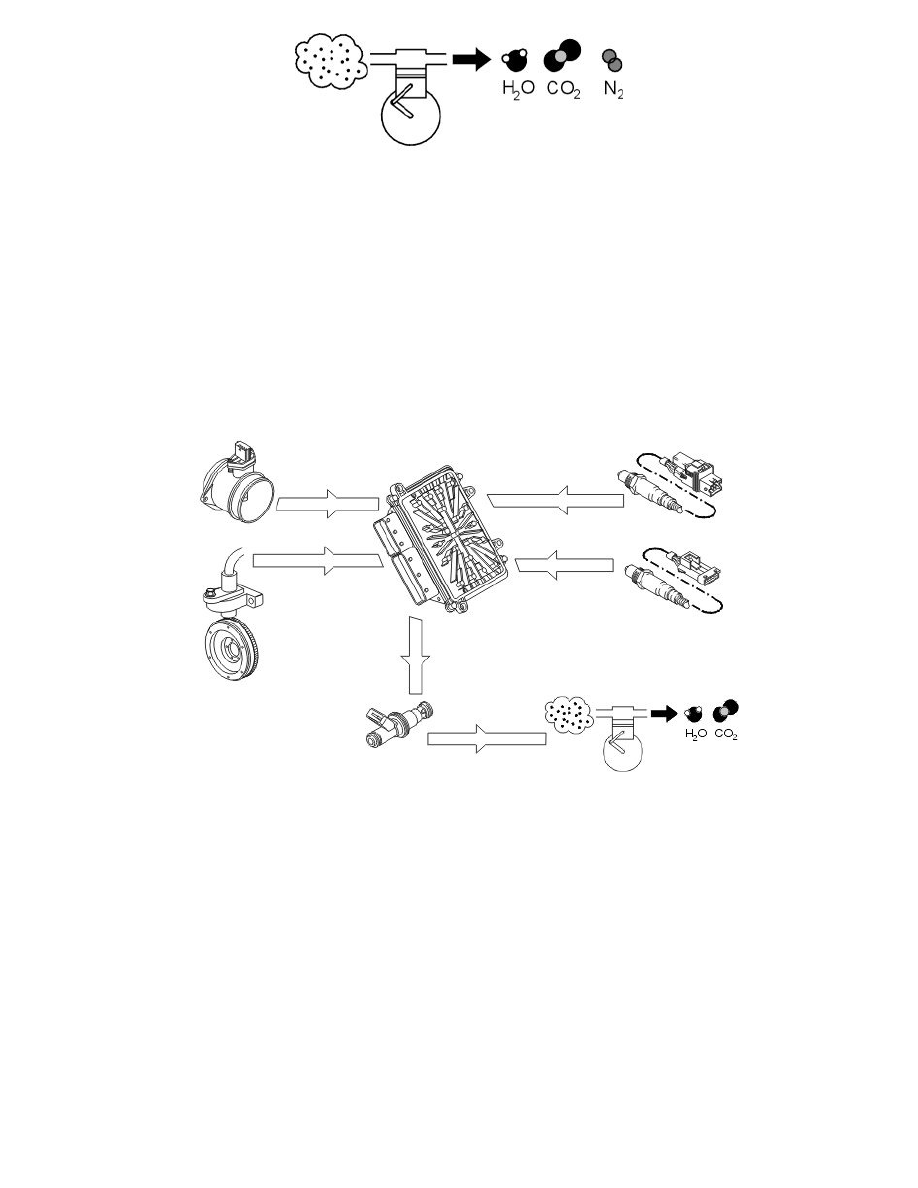Volvo Workshop Manuals

< PREV PAGE NEXT PAGE >
Page 13By speeding up the reaction between the remaining reactive components using a catalytic converter, these can be converted to water (H2O), carbon
dioxide (CO2) and nitrogen (N2).
However this can only happen if the balance of hydro-carbons (HC), carbon monoxide (CO), oxygen (O2) and nitrous oxides (NOx) is exactly right in
the exhaust. This happens when the fuel air mixture before combustion is 14.7 kg of air per kg of fuel. The Lambda value is then said to be one,
(lambda=1).

A base program in the engine control module (ECM) calculates the injection period based on data about load, i.e. the measured air mass and engine
speed (rpm). The calculated injection time (from the base program) is then modified by a circuit (short-term fuel trim). The signal from the heated
oxygen sensor (HO2S) is used to finely adjust the injection period so that lambda=1 is reached. The short-term fuel trim is also a circuit that finely
adjusts the injection period so that the fuel air mixture is optimized (lambda=1). The control module also used the signals from the front and rear heated
oxygen sensors (HO2S) to correct the front heated oxygen sensor (HO2S) (offset adjustment) and thereby the injection period. This gives a higher degree
of accuracy during fuel trim. Fuel trim is a rapid process which may take place several times a second. Adjustment of the calculated injection period
calculated in the base program is limited.
The integrator can be read using VIDA.